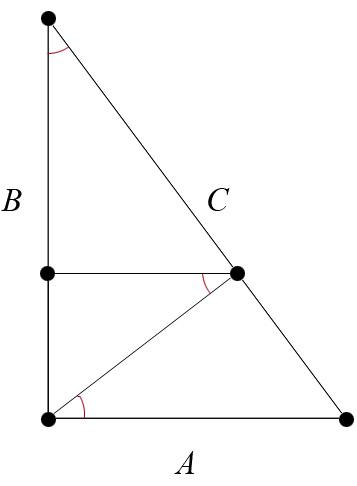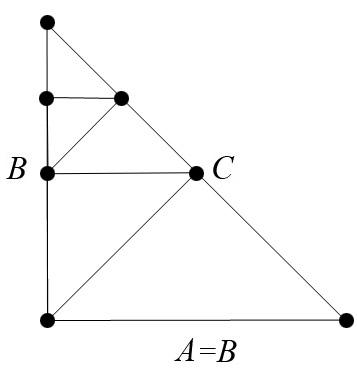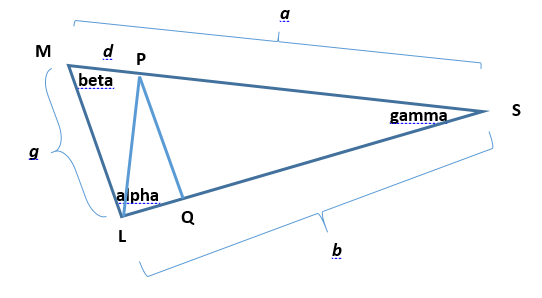BrainDen.com - Brain Teasers
• 0

# Partitioning a Triangle into similar pieces

## Recommended Posts

• 0

 That is what my follow up question was getting at.  But in a 90 degree triangle, where a>b>c, are the three similar triangles restricted in that only certain triangles can be produced?

 ...side note,  how did my follow question drop below bonanova's when my question was asked earlier?

 Yes, I think so. Dissection lines must join an existing vertex to an existing side at a right angle. The process can be continued an infinite number of times, because a self-similar figures is reached after each group ot two lines. This says the first two cuts determine the size ratios, and those ratios remain fixed. Simple inspection will show the ratios are determined by the size of the acute angle..

I don't know of a dissection scheme for non-right triangles.

BTW, and I'm sure this is obvious, I only had to mark ONE of the acute angles as being preserved, since the other acute angle is its complement.

 There are some anomalies in the new version. I'm discussing this one with Site Admin at present. It happened in other threads as well. Meantime check the post dates to be determine the right sequence. To doubt, use the "Quote" feature, as I did here.

##### Share on other sites

• 1

I think this does it.

Construct a right triangle with sides A < B < C and erect perpendiculars on C and B as shown:Three right triangles of unequal size but equal marked acute angles are formed. Having equal angles but unequal size, all four triangles are similar but no two of them are congruent.

##### Share on other sites

• 1

I don't have spoilers on mobile interface, sorry.

Does the problem state ANY triangle?

I can do this for right triangles (which are not isosceles) with 3 similar non congruent triangles and I can do it for equilateral triangles with 3 trapezoids similar non-congruent. But not for any triangle.

EDIT: just saw Bonanova's that is my solution as well, but it does not work for A=B.

Edited by araver
##### Share on other sites

• 1

I share a raver's question about choosing a triangle to dissect. I think only certain triangles are amenable.

##### Share on other sites

• 0

That is what my follow up question was getting at.  But in a 90 degree triangle, where a>b>c, are the three similar triangles restricted in that only certain triangles can be produced?

But yes, my op is for ANY triangle.

I woulf love to see your trapezoid solution araver.

...side note,  how did my follow question drop below bonanova's when my question was asked earlier?

##### Share on other sites

• 0

I can do this for right triangles (which are not isosceles)

EDIT: just saw Bonanova's that is my solution as well, but it does not work for A=B.If it

If it's not obvious why the isosceles case fails ...

You can do the construction exactly the same way to any number of smaller triangles, but the last two triangles will always be congruent.##### Share on other sites

• 0

Purely a hypothesis but i bet we can show that only certain triangles can produce other similar triangles using the fact that for each triangle one of its angles must meet with the other two at a point giving a+b+c=180.  While the sides maintain a proportional relationship.   This sounds like we can create functions and then maybe prove the limitations of the angles...idk

##### Share on other sites

• 0

Sorry, but I was wrong about my "trapezoid" solution. At most two trapezoids would be similar, forcing the third to be similar would make all congruent.

I've circled around and am pretty sure now there's no other way to split a triangle into 3 similar yet non-congruent triangles unless it's follows Bonanova's solution.

And I think the same hypothesis holds for showing no 3 simlar quadriterals can be obtained either (limit case is 3 congruent trapezoids for an equilateral triangle).

With more than 4 sides ... that's even more unlikely, as they won't have the necessary number of sides to begin with (i.e. partition a triangle into 3 pentagons / hexagons).

##### Share on other sites

• 0

Here's my attempt at a proof that only right triangles are solutions to the OP.

Imagine a triangle with three different angles alpha > beta > gamma.

First, let's look at the smallest angle, gamma. No dissection line can split that angle into yet smaller angles, as the resulting triangles have to have the same angles as the original.

There are two ways a line segment can complete the smaller triangle containing gamma, one where the smaller triangle is a smaller mirror image, and one where the smaller triangle is a shadow (ie. long side overlays long side of larger triangle).

The latter case is met by drawing one line parallel to the opposite side.

The enclosed picture shows a scalene triangle MLS with angles alpha (the largest), beta (the middle) and gamma (the smallest).

Construct a parallel PQ to ML in such a way that PLM forms a triangle similar to SML. Here's how: find point P such that length d satisfies a:g = g:d, and draw the parallel there. This length d = g^2/a. Because MLS and PQS have the same angles, they are similar.

Since MLS and MPL share angle beta, and the ratios of the corresponding sides are equal, they are similar.

The tough question is: is PLQ also similar to the others?
* Consider angle LPM. It must be alpha. QPS is beta, so LPQ is gamma, as it is 180-alpha-beta.
* Consider MLP. It must be gamma.
For triangle LPQ to be similar to the others, angle PLQ must be beta.

So this poses a constraint on amenable triangles. This construction will only work if angle alpha = beta plus gamma.

since alpha + beta + gamma = 180, and alpha = beta + gamma, 2* alpha = 180. So this will only work for a right triangle.

This concludes the approach of making PQS similar to MLS by virtue of angle QPS being beta.

Now, the other approach involves making angle QPS = alpha.

The second figure shows line PQ drawn at an arbitrary location. If we connect L and P, this not-to-scale image makes an obviously acute triangle LMP, which could not be similar to the (obtuse) SLM. So let's try the other way of triangulating the quadrangle MLQP, namely by drawing MQ. Since we are subdividing the middle angle beta, the only way similar triangles could result would be if it is divided into two copies of gamma. In other words, the construction would only work if beta = 2*gamma.

This would require angle LQM to be beta (as its other two angles are alpha and gamma).
angle PQS was constructed to be beta, so

PQM must be 180-2*beta.
Angle QPM = 180 - gamma - (180-2*beta) = 2*beta-gamma.

If this triangle is similar to the others, one of these two must be beta, and the other alpha

Either ( 1 ) (PQM = alpha and QMP = beta) or ( 2 ) (PQM = beta and QPM = alpha)
case ( 1 ) : beta = 2*beta - gamma
implies beta = gamma, but that contradicts the constraint from above, that beta = 2*gamma
case ( 2 ) : beta = 180-2*beta, beta = 60
gamma = 30
alpha = 90

In either case, the only triangles amenable to this partition were right angles.oops, sorry, don't know how to attach the files INSIDE the spoiler...

.

Edited by CaptainEd
##### Share on other sites

• 0

Did this prove that it only works on 45-45-90 and 30-60-90 triangles or for all right triangles?

##### Share on other sites

• 0

Neither. It doesn't prove that the condition can be met for right triangles, only that IF the condition can be met at all, it can only be for right triangles.

Edited by CaptainEd
##### Share on other sites

• 0

My English skills are missing the subtlety of what you are saying. Please, if you don't mind, can you you elaborate.

Neither. It doesn't prove that the condition can be met for right triangles, only that IF the condition can be met at all, it can only be for right triangles.

##### Share on other sites

• 0

Bonanova showed that right triangles could be partitioned into similar but non-congruent triangles. All I showed is that no other triangles can be partitioned into similar triangles.

## Join the conversation

You can post now and register later. If you have an account, sign in now to post with your account.×   Pasted as rich text.   Paste as plain text instead

Only 75 emoji are allowed.

×   Your previous content has been restored.   Clear editor

×   You cannot paste images directly. Upload or insert images from URL.